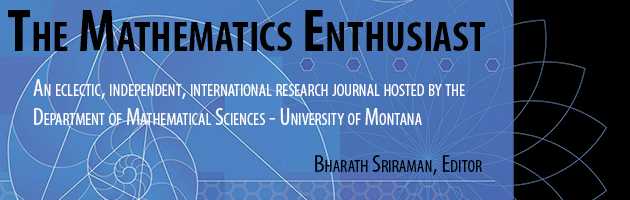•
•#### Article Title

MATHEMATICAL CURIOSITIES ABOUT DIVISION OF INTEGERS

6

3

#### Abstract

As mathematics educators, our focus of attention is mainly placed on the learning and teaching of mathematics. But, as we study phenomena of mathematical learning and teaching, we often come across intriguing mathematical phenomena that capture our interest. We find ourselves often bouncing mathematical ideas back and forth, not just looking for (new/better) ways of teaching or presenting a mathematical concept, but also of uncovering and discovering potential understandings of the concept. These mathematical issues we encounter represent for us a significant aspect of our work, and are also very stimulating. One of these issues arose for us as we were tackling issues of division of numbers and of conventions relating to the remainder; issues that are, mathematically speaking, as we hope to communicate, very interesting and thought provoking. Thus, we explore four different avenues/curiosities about division, where operations with positive and negative numbers are considered, as well as the meaning one can draw out of these operations.

411

422

COinS